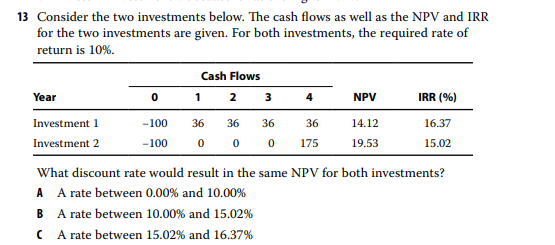# Please may I know some explanation of how to get to the answer by CFA reading 28 EOC Q13The answer simply goes : B is correct. For these investments, a discount rate of 13.16% would yield the
same NPV for both (an NPV of 6.73).

I thought that investment 1 IRR has to reduce so that NPV will increase, and investment 2 IRR has to increase and NPV will decrease, so that the NPV might meet half way, but of course this is wrong. May I know what’s the detailed solution steps are as opposed to the simple solution provided by CFA? Thanks so much in advance

Take the difference between the CF’s and solve for the IRR. Take a wild guess what it is.

1 Like

Thanks so much for your useful response!
May I ask in what context are we taking the difference of the two equations?
Is it essentially a ‘2 equations 2 unknowns’ problem or there’s some other rationale going on?

Edit: Yes. i guess so. Differencing away the same NPV (they are zero anyway for the calculation of IRR) will back out the IRR. Thanks!It’s basically one equation and one unknown. We set the NPV of investment 1 equal to the NPV of investment 2 using the unknown interest rate. A little algebra gets us to discounting the difference in CFs at the unknown interest rate for an initial investment of 0. Solving for that IRR gets you to 13.16%.

The solution could have used a little more detail.

1 Like

That is very helpful. Thank you.